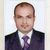# QlikView App Dev

Discussion Board for collaboration related to QlikView App Development.

Announcements
QlikWorld 2023, a live, in-person thrill ride. Save \$300 before February 6: REGISTER NOW!
cancel
Showing results for
Did you mean:Master

## error in exp

Hi all,

currently my variable value is

vCurrentWeekk=Week(today())

so in  expression I used    :  =Count({<WeekNumber={\$(=vThisWeek)}>}id)     this will only only  this week data if I select other week in  filter it is not reflecting   so I tried below expression but its giving error

=if(GetSelectedCount()=0,week(today(),max(WeekNumber)))

1 Solution

Accepted SolutionsMVP

create variable on front end with below expression

=if(GetSelectedCount(Week)=0,week(today()),max(WeekNumber))

now try this expression

=Count({<WeekNumber={"\$(=vThisWeek)"}>}id)

or

=Count({<WeekNumber={"\$(vThisWeek)"}>}id)

Update: Error corrected in variable expression

13 RepliesMVP

Try this

=Count({<WeekNumber={ "\$(=Max(WeekNumber))" }>}id)

=if( GetSelectedCount()=0,week(today() ),max(WeekNumber))) // In this you forget ")"MVP

May be missed parenthesis

=if(GetSelectedCount()=0,week(today()),max(WeekNumber))

Before develop something, think If placed (The Right information | To the right people | At the Right time | In the Right place | With the Right context)Master
Author

still error in expressionMaster
Author

still same errorMVP

Actually this is correct statement

=Count({<WeekNumber={ "\$(=Max(WeekNumber))" }>}id)

Or

=IF( GetSelectedCount()=0, Week(Today()), Max(WeekNumber))Master
Author

still the error in expressionMVP

May be this? Try and check

=Count({<WeekNumber={'\$(=Week(Today()))'}>}id)

Before develop something, think If placed (The Right information | To the right people | At the Right time | In the Right place | With the Right context)MVP

create variable on front end with below expression

=if(GetSelectedCount(Week)=0,week(today()),max(WeekNumber))

now try this expression

=Count({<WeekNumber={"\$(=vThisWeek)"}>}id)

or

=Count({<WeekNumber={"\$(vThisWeek)"}>}id)

Update: Error corrected in variable expressionMVP

Try this as well

=Count( {<WeekNumber={ "\$(=Max(WeekNumber))" }>} id )

Or

=Count( {<WeekNumber={ '\$(=Max(WeekNumber))' }>} id )

Or

=IF( GetSelectedCount()=0, Week(Today()), Max(WeekNumber))

Or

=IF( GetSelectedCount() = IsNull(), Week(Today()), Max(WeekNumber))Community Browser